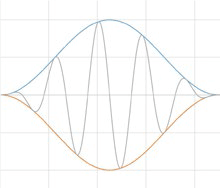Back to Articles

# Why and How Window Functions Are Used

#### Analysis

A signal processing algorithm moves data from the time-domain into the frequency-domain by performing a series of Fast Fourier Transform (FFT) calculations for equally sized time periods, called frames. The underlying FFT mathematics assumes the signals have an infinite, circular topology. The two endpoints of the time waveform are interpreted as though they were connected together, i.e., the endpoints having the same value at each end of a time interval. This is not true for a real-world random signal and the resulting discontinuities in the time domain create unwanted noise in the frequency spectrum, referred to as spectral leakage.

Window functions are extra math added to a signal processing algorithm that addresses, but does not totally overcome, this discontinuity problem. These functions operate by multiplying the time waveform by a finite-length window with an amplitude that varies smoothly and gradually toward zero at the edges. However, note that this multiplication in time results in a distortion in frequency. That is, while the FFT of the windowed waveform will be similar to the FFT of the original waveform, it will have been altered (distorted).Applying a window function

Generally speaking, for a given window function, the larger the window in time (the more FFT analysis lines), the less distortion in frequency. This conflict is summed up by the celebrated Heisenberg Uncertainty Principle: increasing resolution in time (using a smaller window to zoom in on some particular interval of interest) results in decreasing resolution in frequency (more distortion), while decreasing resolution in time (using a larger window) results in increasing resolution in frequency (less distortion).

For a given window size (for a given number of analysis lines), different window functions will result in different distortion characteristics. All window functions are well defined in both time and frequency. The exact characteristics of the resulting distortion are very much dependent on the exact definition of the selected window function.

Mathematicians and data scientists have developed a range of window functions. See the following lessons to learn more about the characteristics that are used to describe these various functions and how they are differentiated from each other.

Date

May 23, 2019

Author

Jared Van Baren

Category